This resource function is obsolete. Use the paclet Wolfram/QuantumFramework instead.

Function Repository Resource:

# QuantumPartialTrace

Partially trace out specified subsystems of a quantum basis, state or operator

Contributed by: Jonathan Gorard
 ResourceFunction["QuantumPartialTrace"][QuantumBasis[…],order] returns the specified QuantumBasis object with the subsystems indexed by order traced out. ResourceFunction["QuantumPartialTrace"][QuantumDiscreteState[…],order] returns the specified QuantumDiscreteState object with the subsystems indexed by order traced out. ResourceFunction["QuantumPartialTrace"][QuantumDiscreteOperator[…],order] returns the specified QuantumDiscreteOperator object with the subsystems indexed by order traced out. ResourceFunction["QuantumPartialTrace"][QuantumMeasurementOperator[…],order] returns the specified QuantumMeasurementOperator object with the subsystems indexed by order traced out. ResourceFunction["QuantumPartialTrace"][QuantumHamiltonianOperator[…],order] returns the specified QuantumHamiltonianOperator object with the subsystems indexed by order traced out. ResourceFunction["QuantumPartialTrace"][QuantumCircuitOperator[…],order] returns the (operator representation of the) specified QuantumCircuitOperator object with the subsystems indexed by order traced out.

## Details

ResourceFunction["QuantumPartialTrace"] will return a QuantumBasis object if its first argument is a QuantumBasis object, a QuantumDiscreteState object if its first argument is a QuantumDiscreteState object, etc. The only exception to this rule is the QuantumCircuitOperator object, whose partial trace is always a QuantumDiscreteOperator object (i.e. ResourceFunction["QuantumPartialTrace"] always returns the operator representation of the resulting circuit).
ResourceFunction["QuantumPartialTrace"] traces out the subsystems in the list order from left to right; therefore, by convention, the list of subsystem indices should be given in descending order.
ResourceFunction["QuantumPartialTrace"], when applied to a QuantumDiscreteState, QuantumDiscreteOperator, QuantumMeasurementOperator, QuantumHamiltonianOperator or QuantumCircuitOperator object, will compute the ResourceFunction["QuantumPartialTrace"] of their associated QuantumBasis objects implicitly.
ResourceFunction["QuantumPartialTrace"][,{}] simply returns its first argument (or its operator form, in the case of circuits) without modification.

## Examples

### Basic Examples (1)

Partially trace out qubits 5, 3 and 1 from a five-qubit Pauli-X QuantumBasis object to obtain a two-qubit QuantumBasis object:

 In:=Out=In:=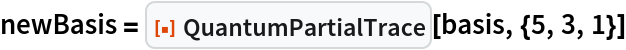Out=In:=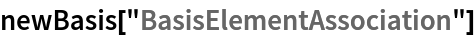Out=### Scope (7)

Partially trace out qubits 3 and 2 from a three-qubit pure QuantumDiscreteState object to obtain a single-qubit pure QuantumDiscreteState object:

 In:=Out=In:=Out=In:=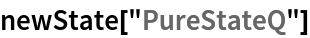Out=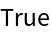On the other hand, if we partially trace out qubits 3 and 1 instead, then we obtain a single-qubit mixed QuantumDiscreteState object:

 In:=Out=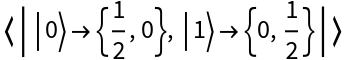In:=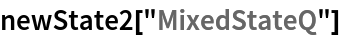Out=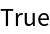In:=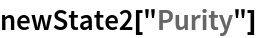Out=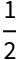Partially trace out qubit 2 from a two-qubit mixed QuantumDiscreteState object to obtain a single-qubit mixed QuantumDiscreteState object:

 In:=Out=In:=Out=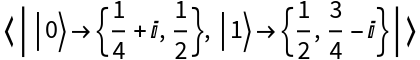In:=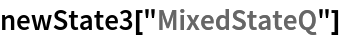Out=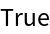On the other hand, if we partially trace out qubit 1 instead, then we obtain a single-qubit pure QuantumDiscreteState object:

 In:=Out=In:=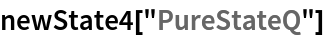Out=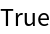Partially trace out qubit 1 from an arity-3 QuantumDiscreteOperator object to obtain an arity-2 QuantumDiscreteOperator object:

 In:=Out=In:=Out=In:=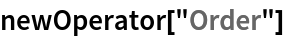Out=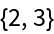Partially trace out qubits 2 and 1 instead, to obtain an arity-1 QuantumDiscreteOperator object:

 In:=Out=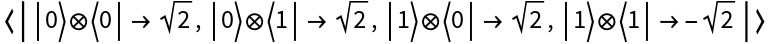In:=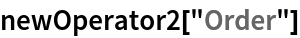Out=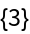Partially trace out qubit 4 from an arity-4 projection-valued QuantumMeasurementOperator object to obtain an arity-3 QuantumMeasurementOperator object:

 In:=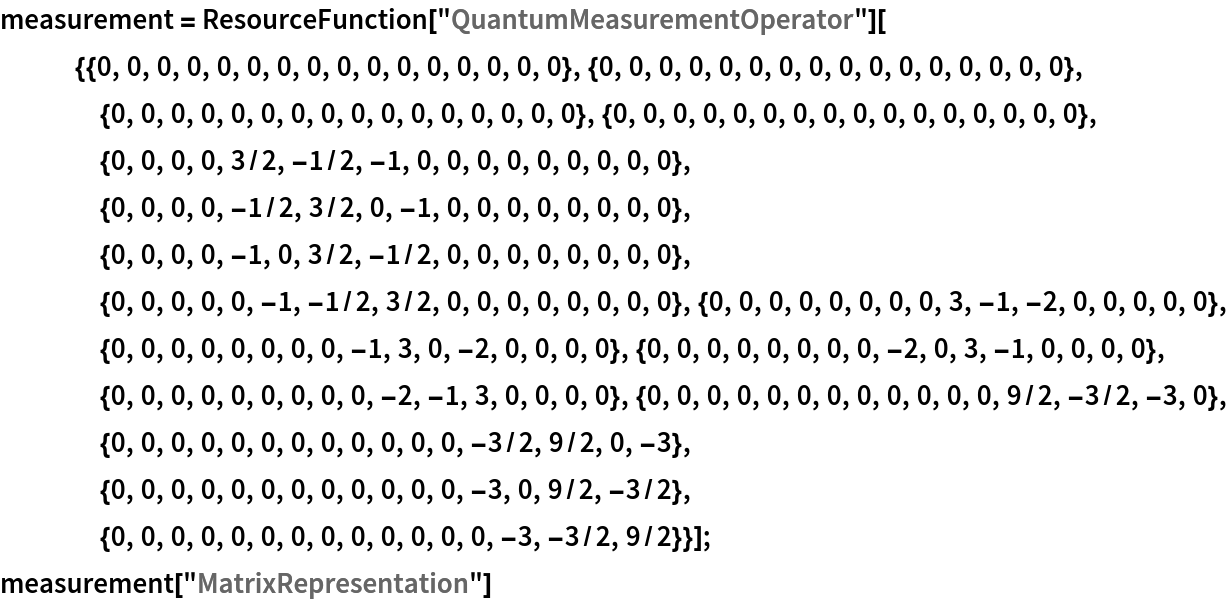Out=In:=Out=In:=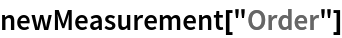Out=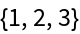Partially trace out qubits 3 and 2 instead, to obtain an arity-2 QuantumMeasurementOperator object:

 In:=Out=In:=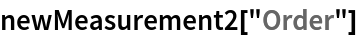Out=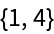Partially trace out qubit 2 from an arity-2 positive operator-valued QuantumMeasurementOperator object to obtain an arity-1 positive operator-valued QuantumMeasurementOperator object:

 In:=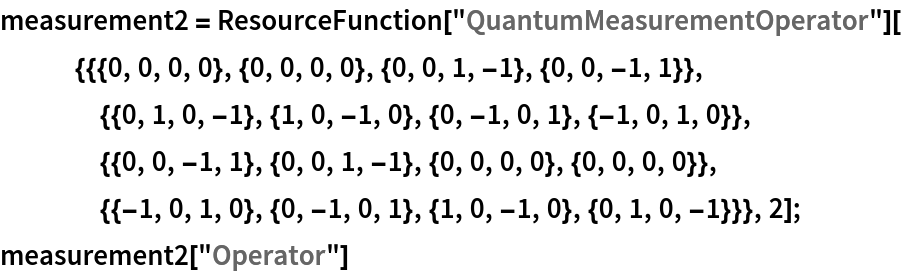Out=In:=Out=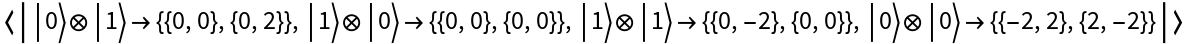Partially trace out qubit 1 instead:

 In:=Out=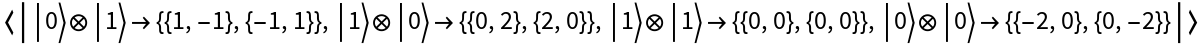Partially trace out qubit 1 from an arity-2 QuantumHamiltonianOperator object to obtain an arity-1 QuantumHamiltonianOperator object:

 In:=Out=In:=Out=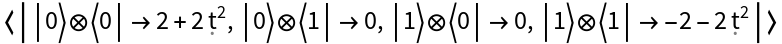Partially trace out qubit 2 instead:

 In:=Out=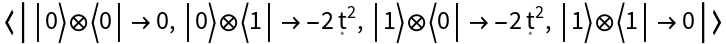Partially trace out qubit 3 from an arity-3 QuantumCircuitOperator object to obtain an arity-2 QuantumDiscreteOperator object:

 In:=Out=In:=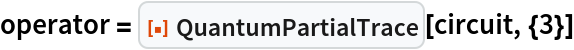Out=In:=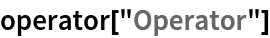Out=Partially trace out qubits 3 and 1 instead, to obtain an arity-1 QuantumDiscreteOperator object:

 In:=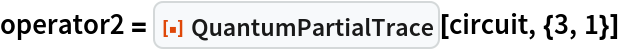Out=In:=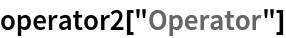Out=Partially trace over higher-dimensional quantum objects:

 In:=Out=In:=Out=In:=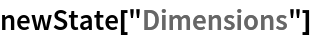Out=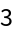When taking the partial trace of a QuantumDiscreteState, QuantumDiscreteOperator, QuantumMeasurementOperator, QuantumHamiltonianOperator or QuantumCircuitOperator object, QuantumPartialTrace will also compute the partial trace of the associated QuantumBasis objects implicitly:

 In:=Out=In:=Out=In:=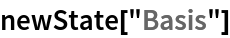Out=The resulting basis is equivalent to partially tracing over the original basis:

 In:=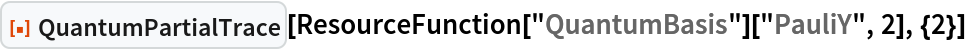Out=Jonathan Gorard

## Version History

• 1.0.0 – 02 June 2021